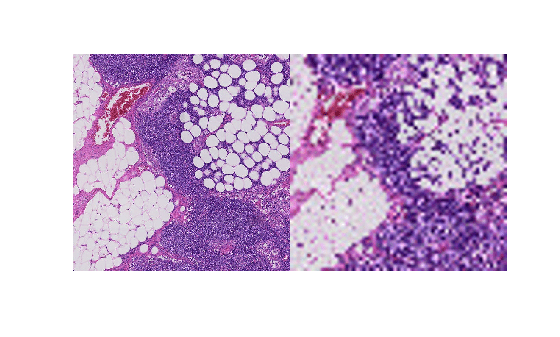# getRegion

Read arbitrary region of blocked image

Since R2021a

## Syntax

``pixels = getRegion(bim,pixelStart,pixelEnd)``
``pixels = getRegion(bim,pixelStart,pixelEnd,Level=L)``

## Description

example

````pixels = getRegion(bim,pixelStart,pixelEnd)` returns all the pixels in the blocked image `bim`, in the region specified by `pixelStart` and `pixelEnd`. ```
````pixels = getRegion(bim,pixelStart,pixelEnd,Level=L)` gets the specified region from the `L`'th resolution level of the multiresolution `blockedImage` object, `bim`. `pixelStart` and `pixelEnd` are pixel subscripts at the `L`'th resolution level.```

## Examples

collapse all

Create a blocked image.

`bim = blockedImage("tumor_091R.tif");`

Specify the start and end points of a region in the blocked image at resolution level 1, and retrieve the data.

```pixelStartLevel1 = [2100 1800]; pixelEndLevel1 = [2600 2300]; imLevel1 = getRegion(bim,pixelStartLevel1,pixelEndLevel1,Level=1);```

Convert the start and end points of the region from pixel subscripts to world coordinates.

```worldStartLevel1 = sub2world(bim,pixelStartLevel1,Level=1); worldEndLevel1 = sub2world(bim,pixelEndLevel1,Level=1);```

Convert the start and end points of the region from world coordinates to pixel subscripts at resolution level 3, and retrieve the data.

```pixelStartLevel3 = world2sub(bim,worldStartLevel1,Level=3); pixelEndLevel3 = world2sub(bim, worldEndLevel1,Level=3); imLevel3 = getRegion(bim,pixelStartLevel3,pixelEndLevel3,Level=3);```

Display the region at both resolution levels.

`montage({imLevel1,imLevel3});`## Input Arguments

collapse all

Blocked image, specified as a `blockedImage` object.

Starting pixel of the region at the desired resolution level, specified as a 1-by-N vector of pixel subscripts in the form `[row,column,...,subN]`. N is equal to or less than the number of dimensions of the blocked image `bim`. If you specify pixel subscripts with a smaller dimensionality than the blocked image, then the `getRegion` function pads the remaining dimensions with the value `1`.

Ending pixel of the region at the desired resolution level, specified as a 1-by-N vector of pixel subscripts in the form `[row,column,...,subN]`. N is equal to or less than the number of dimensions of the blocked image `bim`. If you specify pixel subscripts with a smaller dimensionality than the blocked image, then the `getRegion` function pads the remaining dimensions with the value `1`.

Resolution level, specified as a positive integer that is less than or equal to the number of resolution levels of `bim`.

## Output Arguments

collapse all

Pixels in specified region, returned as a numeric array.

## Version History

Introduced in R2021a

expand all